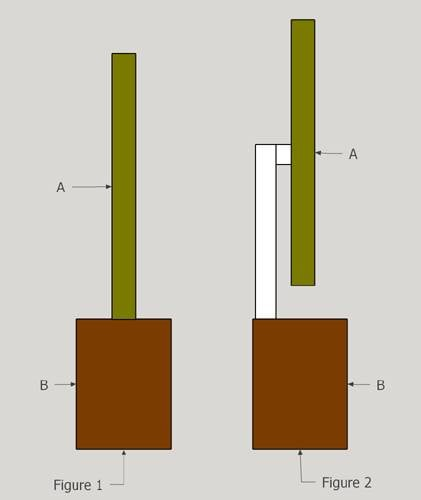# Weight distribution of 2 configurationsSay Object-A weighs 100 lbs.
In Figure-1, it's obvious that the weight distribution is even along the bottom edge of Rectangle-B.

In Figure-2, ignoring the weight of the white objects, is the weight distribution exactly the same along the bottom edge of Rectangle-B as it is in Figure-1?

Mentor
What do you think, and why? Are you familiar with how to draw Free Body Diagrams (FBDs) and sum forces and moments?

Summary:: Weight distribution

View attachment 253509

Say Object-A weighs 100 lbs.
In Figure-1, it's obvious that the weight distribution is even along the bottom edge of Rectangle-B.

In Figure-2, ignoring the weight of the white objects, is the weight distribution exactly the same along the bottom edge of Rectangle-B as it is in Figure-1?
Hint: The moments around the center of mass must balance in both cases.

What do you think, and why? Are you familiar with how to draw Free Body Diagrams (FBDs) and sum forces and moments?
Nope. I'm just a curious senior. This is not a homework problem.

Hint: The moments around the center of mass must balance in both cases.
You totally lost me. I'm just a curious senior hoping for an explanation from the gurus.

Mentor
You totally lost me. I'm just a curious senior hoping for an explanation from the gurus.
It's good to be curious. Google "sum of moments" and "free body diagram".

Senior in high school?It's good to be curious. Google "sum of moments" and "free body diagram".

Senior in high school?Nope, senior as in 79 years old.

Mentor
Nope, senior as in 79 years old.
Ah, got it, LOL.

What is your math background? It doesn't take advanced math to work out a sum of moments (a moment is basically a torque, multiplying the force by the lever arm length, and ratioed to the angle that the force is applied at the end of the lever arm).

Here is a basic article about FBDs:

https://en.wikipedia.org/wiki/Free_body_diagram

And here are the Google search results for Sum of Moments:

which includes a couple instruction videos on how to apply the sum of moments to solve a statics problem like the one you have asked about.•anorlunda
Mentor
Summary:: Weight distribution

View attachment 253509

Say Object-A weighs 100 lbs.
In Figure-1, it's obvious that the weight distribution is even along the bottom edge of Rectangle-B.
This is not correct. The force distribution along the bottom edge of rectangle B in Figure 1 is not uniform. It is higher toward the center than towards the edges.

Have you ever had a course in calculus? If you are talking about the force distribution along the bottom edge, are you comfortable with the idea that the force distribution is described by the local value of the upward force per unit width?

•berkeman
This is not correct. The force distribution along the bottom edge of rectangle B in Figure 1 is not uniform. It is higher toward the center than towards the edges.

Have you ever had a course in calculus? If you are talking about the force distribution along the bottom edge, are you comfortable with the idea that the force distribution is described by the local value of the upward force per unit width?
Let me reword my statement.

"In Figure-1, if 2 small blocks of wood are placed underneath Rectangle-B, one flush with the left edge and the other flush with the right edge, then the weight pressing on each block would be identical assuming the load is directly in the center of Rectangle-B."

Will the same condition exist in Figure-2?

Mentor
Will the same condition exist in Figure-2?
What do you think and why? (Have you actuially been reading the links and hints we have been giving you? )

Yes, I googled the suggestions but could not figure out the answer to my question. That's OK. I didn't come here for a physics lesson. I should have known better having read replies to questions from other people. I just hoped for an answer but it's like pulling teeth.

I understand that gurus here don't want to give answers. Only to guide people to figuring out the answers for themselves. No problem with that. It's just not my goal at 79 years.

Bye physicsforum. There's no point in my returning.

Mentor
I understand that gurus here don't want to give answers. Only to guide people to figuring out the answers for themselves. No problem with that. It's just not my goal at 79 years.
Thanks for the complement. That is indeed what we try to do. Best wishes.

•